<img alt="" src="https://secure.perk0mean.com/171547.png" style="display:none;">

# Using Microsoft Excel to write SAS code in Clinical TrialsOften when we write SAS code in the pharmaceutical industry, there is a high level of repetition. This guide explains ways of writing repetitive SAS code using Excel that will reduce the overall time to write the code and make large scale amendments easier and quicker.

#### The attrib statement

One way of specifying the attributes of a new or existing variable in SAS is to use the attrib statement. The attrib statement can be used to set the name, length, type, format and label of a field. Obviously, writing an attrib statement to set these attributes for every single field in your output dataset would take a long time; this is where Excel can help.

Imagine you have been sent a programming plan that lists one thousand fields with their associated length, type, format and label: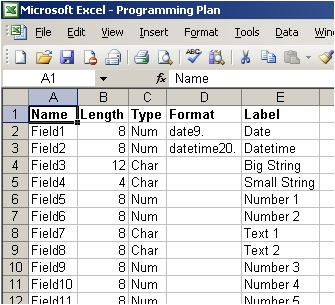Excel has the ability to concatenate strings using the ampersand (&) operator. It is also possible to perform operations during the concatenation.

To create the first attrib statement we can put the following formula in to cell F2:

="attrib "&;LOWER(A2)&" length = "&IF(C2="Char","\$","")&B2&IF(D2<>""," format = "&D2,"")&" label = '"&E2&"';"

This formula will produce:

attrib field1 length = 8 format = date9. label = 'Date';

Use the colours to see which part of the formula produces each part of the string. Notice that the part in green does not produce anything for this attrib statement. This is because this part of the formula is the part that will include a “\$” to denote a character field, and the current example is numeric.

The cell F2 can now be filled down by selecting the cell and double clicking on the bottom right-hand corner of the selection box. This has the same effect as copying F2 and pasting it in to every other cell in column F where there is a non blank cell in column E for the same row. (This is how the fill down method knows where to stop).

Top tip
When you copy and paste a cell that contains an unlocked formula the formula is adjusted so the source cells become the equivalent relation of the source cells to the cell being copied. For example: copying cell A1 that contains the formula =B1 in to cell A2, would change the formula to =B2. To prevent this from happening references to source cells can be locked using the \$ character. For example: copying cell A1 that contains the formula =\$B\$1 in to any other cell would not affect the formula. The row and column references can also be locked independently.

Once cell F2 has been filled down, we are left with: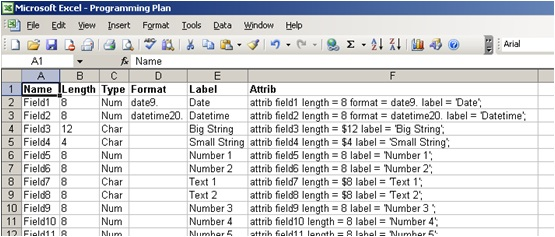These resulting attrib statements can be copied straight in to a data step in your SAS code.

#### Macro Calls

One of the fastest ways to write SAS code that does a similar task many times over is to use the SAS macro language. Unfortunately once your macro has been written, you also need to write the macro invocations, each with specific parameters that will amend the SAS code that is executed during each invocation. Excel can be used to write these macro invocations in a similar way to the attrib statements described above.

The programming plan for our one thousand variable dataset also contains the criteria to be used to derive each one of the fields.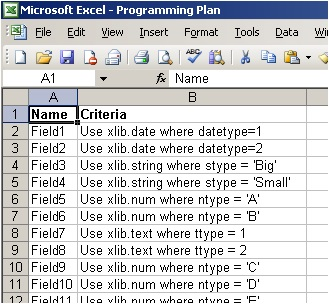As the criteria for each field is similar, we could use the following macro to derive every field:

%macro excel_example(field, source, where);

data &field;
set &source (keep=subjid &field);
where &where;
run;

%mend;

The macro needs three parameters to derive the fields: field (field name), source (source dataset) and where (where clause).

In order to construct our macro invocation we first need to extract the parameters required from the criteria in the programming plan. The best way to do this is to work out what comes before and after the parts of the criteria we want to extract and use the Excel MID( ) function to extract them.

Source:
The source dataset and library appear after the first space and before the second space. The first space can be found using:

=SEARCH(" ",B2,1)

Using the result of this formula we can find the second space by incrementing its result by 1 and using this as the starting position for a second SEARCH( ):

=SEARCH(" ",B2,SEARCH(" ",B2,1)+1)

Now we have are start and end point we can use MID( ) to extract the source dataset and library name from the criteria:

=MID(B2,SEARCH(" ",B2,1),SEARCH(" ",B2,SEARCH(" ",B2,1)+1)-SEARCH(" ",B2,1))

This formula can now be filled down by selecting the cell and double clicking on the bottom right-hand corner of the selection box.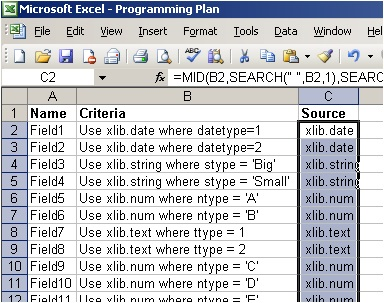Where:
The where clause can be extracted from the criteria in a similar way using the word “where” to determine its starting position, and the length of the criteria string as the end:

=MID(B2,SEARCH("where",B2,1)+6,LEN(B2)-SEARCH("where",B2,1))

Notice the +6 in the middle of the formula, this is used to advance the starting position of the MID function past the word “where“.

This formula can now be filled down to provide the where criteria for the rest of the fields in the programming plan: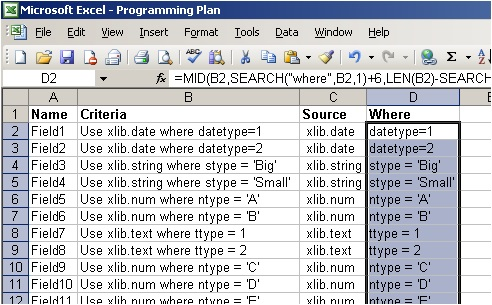Now that the parameters required by our macro have been extracted, the macro invocations can be created by concatenating them together along with the necessary SAS code using the ampersand (&) operator:

="%excel_example("&LOWER(A2)&", "&C2&", %str("&D2&"));"

Will provide:

%excel_example(field1, xlib.date, %str(datetype=1));

As the where criteria are likely to contain SAS special characters that might upset the macro process, the where criteria have been enclosed in the %str macro function that will suppress any special characters until the parameter is called.

This last formula can now be filled down to provide the macro invocation for every field in the programming plan.

Entering comments into SAS code can often be one of the most tedious activities any programmer can undertake. However SAS code that is commented effectively is much easier to maintain and debug than plain SAS code.

By making a small addition to the formula that produces the macro invocations we can put a comment above each macro invocation based on the information contained in the programming plan:

="/*"&A2&" - "&B2&"*/
"&"%excel_example("&LOWER(A2)&", "&C2&", %str("&D2&"));"

Notice that the formula appears on two lines, this is because a carriage return has been inserted after the comment. This is done by placing the cursor at the required point in the formula and pressing return while holding down the Alt key. When the macro invocations are pasted into source code, the carriage return will put the comments and macro invocations on separate lines: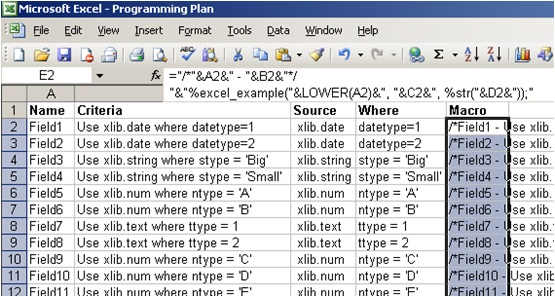%macro excel_example(field, source, where);

data &field;
set &source (keep=subjid &field);
where &where;
run;

%mend;

/*Field1 - Use xlib.date where datetype=1*/
%excel_example(field1, xlib.date, %str(datetype=1));
/*Field2 - Use xlib.date where datetype=2*/
%excel_example(field2, xlib.date, %str(datetype=2));
/*Field3 - Use xlib.string where stype = 'Big'*/
%excel_example(field3, xlib.string, %str(stype = 'Big'));
/*Field4 - Use xlib.string where stype = 'Small'*/
%excel_example(field4, xlib.string, %str(stype = 'Small'));
/*Field5 - Use xlib.num where ntype = 'A'*/
%excel_example(field5, xlib.num, %str(ntype = 'A'));
/*Field6 - Use xlib.num where ntype = 'B'*/
%excel_example(field6, xlib.num, %str(ntype = 'B'));
/*Field7 - Use xlib.text where ttype = 1*/
%excel_example(field7, xlib.text, %str(ttype =1));
/*Field8 - Use xlib.text where ttype = 2*/
%excel_example(field8, xlib.text, %str(ttype = 2));
/*Field9 - Use xlib.num where ntype = 'C'*/
%excel_example(field9, xlib.num, %str(ntype = 'C'));
/*Field10 - Use xlib.num where ntype = 'D'*/
%excel_example(field10, xlib.num, %str(ntype = 'D'));
/*Field11 - Use xlib.num where ntype = 'E'*/
%excel_example(field11, xlib.num, %str(ntype = 'E'));

Running this macro will provide one dataset per field that can then be merged together by the key value (subjid).

#### Tips

F4
Often when working with Excel you need to perform the same task many times over. Once you have performed a task once, pressing the F4 key will repeat the task on any item you select. For example, if you want to highlight alternating rows to make the data easier to look at you can select the first row, right click and choose your format options, click ok. Then subsequent rows need only be selected and F4 pressed to format them in the same way.

Fit to size
Resizing rows and columns can be annoying when you have hundreds of them. If you hover on the line between two column letters until the resize cursor appears and double click, that column will be resized to fit the largest cell in that column. The same works for rows when clicking between two row numbers. The entire sheet can be done at once by selecting all cells before performing this operation.

Don’t waste time
The examples given above are perfect and uniform and none of the formulas used needed to be adjusted for rows that didn’t work properly. In the real world there will always be problems that need to be overcome in using Excel to write code. Try to remember that this method will allow you to do large amounts quickly, but if you spend too long trying to make your formulas work perfectly for every single row then you may end up taking longer than if you had just written the few troublesome lines manually.## Related Articles#### The INTO Statement in PROC SQL to Create Macro Variables

by Clinical Programming Team#### Performing CDISC Compliant Pharmacokinetic Analyses

by Statistical Consultancy Team